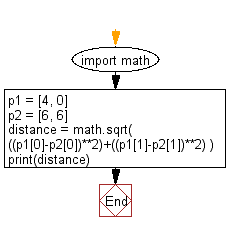﻿ Python: Compute the distance between two points - w3resource# Python: Compute the distance between two points

## Python Basic: Exercise-40 with Solution

Write a Python program to compute the distance between the points (x1, y1) and (x2, y2).

Pictorial Presentation:Sample Solution:-

Python Code:

``````import math
p1 = [4, 0]
p2 = [6, 6]
distance = math.sqrt( ((p1-p2)**2)+((p1-p2)**2) )

print(distance)
```
```

Sample Output:

```6.324555320336759
```

Flowchart:## Visualize Python code execution:

The following tool visualize what the computer is doing step-by-step as it executes the said program:

Python Code Editor:

Have another way to solve this solution? Contribute your code (and comments) through Disqus.

What is the difficulty level of this exercise?

Test your Python skills with w3resource's quiz

﻿

## Python: Tips of the Day

Unpack variables from iterable:

```# One can unpack all iterables (tuples, list etc)
>>> a, b, c = 1, 2, 3
>>> a, b, c
(1, 2, 3)

>>> a, b, c = [1, 2, 3]
>>> a, b, c
(1, 2, 3)
```